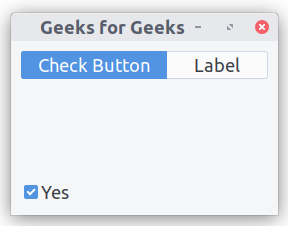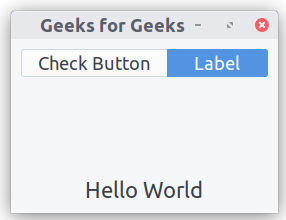Open in App
Not now

# Python – Stack and StackSwitcher in GTK+ 3

• Last Updated : 31 Dec, 2020

A `Gtk.Stack` is a container which allows visibility to one of its child at a time. Gtk.Stack does not provide any direct access for users to change the visible child. Instead, the `Gtk.StackSwitcher` widget can be used with `Gtk.Stack` to obtain this functionality.
In `Gtk.Stack` transitions between pages can be done by means of slides or fades. This can be controlled with `Gtk.Stack.set_transition_type()`.

The `Gtk.StackSwitcher` widget acts as a controller for a `Gtk.Stack` ; it shows a row of buttons to switch between the various pages of the associated stack widget. The content for the buttons comes from the child properties of the `Gtk.Stack`.

1. import GTK+ 3 module.
2. Create main window.
3. Implement Stack.
4. Implement Button.
5. Implement Label.
6. Implement StackSwitcher.
Example :

 `import` `gi ` `# Since a system can have multiple versions ` `# of GTK + installed, we want to make  ` `# sure that we are importing GTK + 3. ` `gi.require_version(``"Gtk"``, ``"3.0"``) ` `from` `gi.repository ``import` `Gtk ` ` `  ` `  `class` `StackWindow(Gtk.Window): ` `    ``def` `__init__(``self``): ` `        ``Gtk.Window.__init__(``self``, title ``=``"Geeks for Geeks"``) ` `        ``self``.set_border_width(``10``) ` ` `  `        ``# Creating a box vertically oriented with a space of 100 pixel. ` `        ``vbox ``=` `Gtk.Box(orientation ``=` `Gtk.Orientation.VERTICAL, spacing ``=` `100``) ` `        ``self``.add(vbox) ` ` `  `        ``# Creating stack, transition type and transition duration. ` `        ``stack ``=` `Gtk.Stack() ` `        ``stack.set_transition_type(Gtk.StackTransitionType.SLIDE_LEFT_RIGHT) ` `        ``stack.set_transition_duration(``1000``) ` ` `  `        ``# Creating the check button. ` `        ``checkbutton ``=` `Gtk.CheckButton(``"Yes"``) ` `        ``stack.add_titled(checkbutton, ``"check"``, ``"Check Button"``) ` ` `  `        ``# Creating label . ` `        ``label ``=` `Gtk.Label() ` `        ``label.set_markup(``"Hello World"``) ` `        ``stack.add_titled(label, ``"label"``, ``"Label"``) ` ` `  `        ``# Implementation of stack switcher. ` `        ``stack_switcher ``=` `Gtk.StackSwitcher() ` `        ``stack_switcher.set_stack(stack) ` `        ``vbox.pack_start(stack_switcher, ``True``, ``True``, ``0``) ` `        ``vbox.pack_start(stack, ``True``, ``True``, ``0``) ` ` `  ` `  `win ``=` `StackWindow() ` `win.connect(``"destroy"``, Gtk.main_quit) ` `win.show_all() ` `Gtk.main() `

Output :My Personal Notes arrow_drop_up
Related Articles